# What is Absolute reference in Excel

In this article, we will learn how to create and use absolute reference in excel.

In simple words, Excel lets you freeze cell reference. When you copy and paste formulas in excel. Dynamic cell reference occurs.

Here you can see cell reference is created. Use the slide down feature to copy formulas. You have to copy the cell formula as text to paste

Note: Slide down feature in excel use the formula in the first cell and paste it up to the cells until you stop sliding down.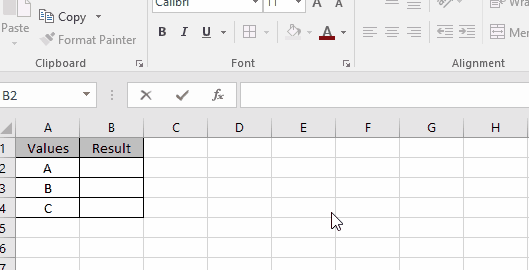As you can see the formula copies corresponding values, not the same value.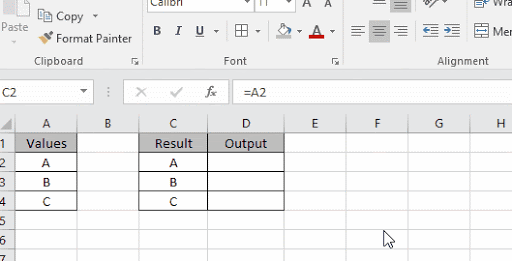Here we copy the formula and paste in the adjacent cell you will notice that cell reference changed.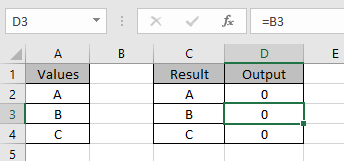As you can see the formulas in A1, A2 & A3 cell are changed. When paste the formula in adjacent ones.

Now we use absolute reference using the (\$)dollar sign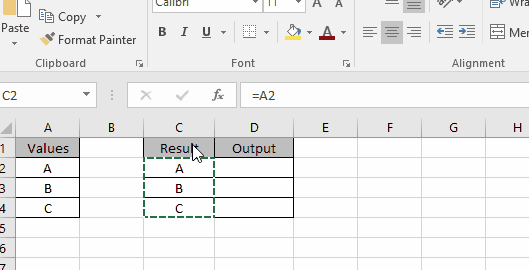You know what I did here. Just use the dollar sign in front of row name if you need to freeze the column or use the dollar sign in front of column name to freeze the row or use the dollar sign in front of both to freeze the cell reference.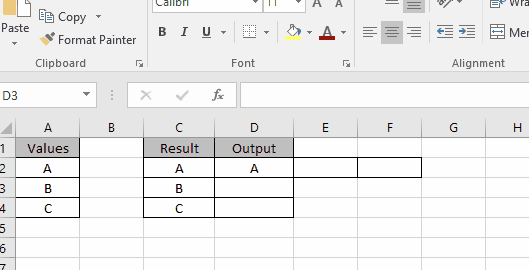Let’s learn this using it an example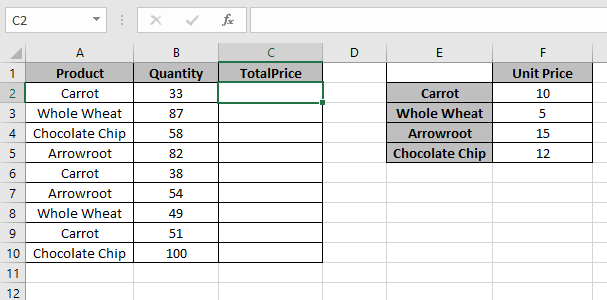Here we need to find totalPrice for all the products.
TotalPrice = Quantity * UnitPrice
Cell reference for quantity should be : \$B2
Cell reference for UnitPrice should be : \$F\$2(For Carrot)

Use the formula for Carrot

=\$B2*\$F\$2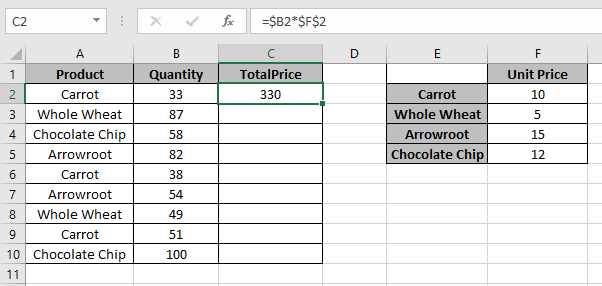Use the formula for each product as we are using absolute cell reference.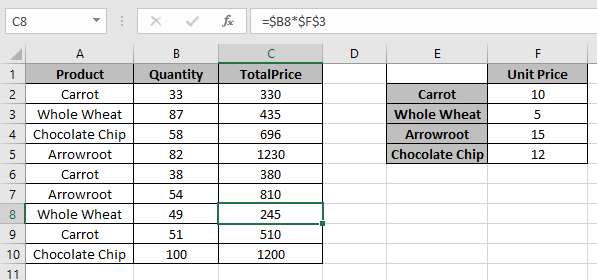As you can see absolute cell reference can help with quick calculation.

Hope you understood How to create and use absolute cell reference in Excel 2016. Find more articles on Excel cell references here. Please share your query below in the comment box. We will assist you.

Related Article:

Relative and Absolute Reference in Excel

Shortcut To Toggle Between Absolute and Relative References in Excel

Dynamic Worksheet Reference in Excel

Expanding References in Excel

Popular Articles:

How to use the VLOOKUP Function in Excel

How to use the COUNTIF function in Excel

How to use the SUMIF Function in Excel

Terms and Conditions of use

The applications/code on this site are distributed as is and without warranties or liability. In no event shall the owner of the copyrights, or the authors of the applications/code be liable for any loss of profit, any problems or any damage resulting from the use or evaluation of the applications/code.